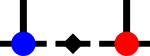SyTen## ◆ getAvgBlocksize()

template<Rank rank>
 Index syten::Tensor< rank >::getAvgBlocksize ( Index leg ) const
inline

get averaged sidelength of blocks as $$\left[\frac{1}{N} \sum_i m_i^3 \right]^{1/3}$$

This is a fairly good measure of computational cost as matrix-matrix multiplication tends to scale with the third power of the blocksize. One block of the resulting size will then likely cost as much as all blocks of the current size.

Referenced by syten::btt_operator_fbasis().Here is the caller graph for this function: